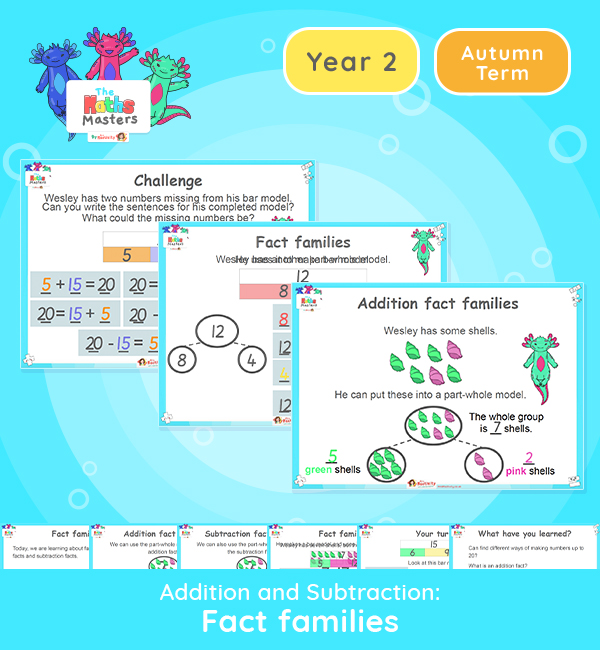# Year 2 | Fact Families to 20 Lesson PresentationAligned with the maths mastery approach, this Year 2 | Fact Families to 20 Lesson Presentation is fully editable, and is designed for the Year 2 maths curriculum covering the following maths objectives for the autumn term:

This lesson focuses on addition and subtraction facts for numbers up to 20.

Small Steps: Addition and subtraction bonds to 20.

NC Links: Recall and use addition and subtraction facts to 20 fluently. • Add and subtract numbers using concrete objects, pictorial representations and mentally. • Show that the addition of two numbers can be done in any order (commutative) and subtraction of one number from another cannot.

Previous Experience: Learn and use numbers bonds to 10. Partition numbers within 10.

TAF Statements: Working Towards: Recall at least four of the six number bonds to 10 and reason about associated facts.

Working At: Recall all the number bonds to and within 10, and use these to reason with and calculate bonds to and within 20, recognising other associated additive relationships.

Aligned with the order of teaching of the White Rose Maths scheme of work, use this to help your class get to grips with each mathematical concept. This lesson presentation also includes varied fluency activities, problem solving, and mathematical discussion questions too.

Explore our other  year 2 addition and subtraction resources.

## Recently Viewed